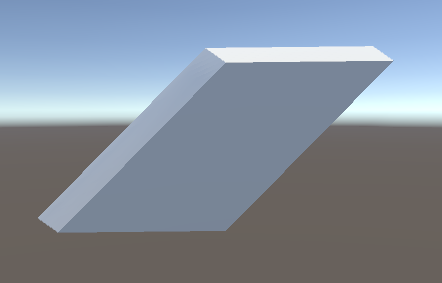# Shear transformation using GameObject transformations

Is it possible to create shear transformation (for 2 or at least 1 axis) using cascade of GameObject transform operations?

I know that this is possible to do using Mesh API or proper shader, but I would like to know if the same effect can be achieved using only plain GameObjects and some scripting instead.

It can be done. Example:

• Outermost game object: Rotation X=22.5, Y=0, Z=0
• Next game object in: Scale X=1, Y=0.3826834, Z=0.9238795
• Next game object in: Rotation X=-45, Y=0, Z=0
• Next game object in: Scale X=1, Y=2, Z=1.414214
• Standard unit cube inside that.

Produces a 45 degree shear on X:The general case:

• Rotate by X=theta/2 counter-clockwise
• Scale by Y=sin(theta/2), Z=cos(theta/2)
• Rotate by X=45 degrees clockwise
• Scale by Y=sqrt(2)/sin(theta), Z=sqrt(2).

You can probably reduce that to two game objects but I left it as 4 to illustrate how many transformations are being done.

No. Transforms can only translate, rotate, and scale. Combining those operations never produces shear. You will have to use something else to produce shear.